# 8.1 Graphs of the sine and cosine functions  (Page 8/13)

 Page 8 / 13

For the equation $\text{\hspace{0.17em}}A\text{\hspace{0.17em}}\mathrm{cos}\left(Bx+C\right)+D,$ what constants affect the range of the function and how do they affect the range?

The absolute value of the constant $\text{\hspace{0.17em}}A\text{\hspace{0.17em}}$ (amplitude) increases the total range and the constant $\text{\hspace{0.17em}}D\text{\hspace{0.17em}}$ (vertical shift) shifts the graph vertically.

How does the range of a translated sine function relate to the equation $\text{\hspace{0.17em}}y=A\text{\hspace{0.17em}}\mathrm{sin}\left(Bx+C\right)+D?$

How can the unit circle be used to construct the graph of $\text{\hspace{0.17em}}f\left(t\right)=\mathrm{sin}\text{\hspace{0.17em}}t?$

At the point where the terminal side of $\text{\hspace{0.17em}}t\text{\hspace{0.17em}}$ intersects the unit circle, you can determine that the $\text{\hspace{0.17em}}\mathrm{sin}\text{\hspace{0.17em}}t\text{\hspace{0.17em}}$ equals the y -coordinate of the point.

## Graphical

For the following exercises, graph two full periods of each function and state the amplitude, period, and midline. State the maximum and minimum y -values and their corresponding x -values on one period for $\text{\hspace{0.17em}}x>0.\text{\hspace{0.17em}}$ Round answers to two decimal places if necessary.

$f\left(x\right)=2\mathrm{sin}\text{\hspace{0.17em}}x$

$f\left(x\right)=\frac{2}{3}\mathrm{cos}\text{\hspace{0.17em}}x$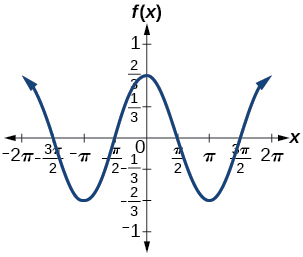amplitude: $\text{\hspace{0.17em}}\frac{2}{3};\text{\hspace{0.17em}}$ period: $\text{\hspace{0.17em}}2\pi ;\text{\hspace{0.17em}}$ midline: $\text{\hspace{0.17em}}y=0;\text{\hspace{0.17em}}$ maximum: $\text{\hspace{0.17em}}y=\frac{2}{3}\text{\hspace{0.17em}}$ occurs at $\text{\hspace{0.17em}}x=0;\text{\hspace{0.17em}}$ minimum: $\text{\hspace{0.17em}}y=-\frac{2}{3}\text{\hspace{0.17em}}$ occurs at $\text{\hspace{0.17em}}x=\pi ;\text{\hspace{0.17em}}$ for one period, the graph starts at 0 and ends at $\text{\hspace{0.17em}}2\pi$

$f\left(x\right)=-3\mathrm{sin}\text{\hspace{0.17em}}x$

$f\left(x\right)=4\mathrm{sin}\text{\hspace{0.17em}}x$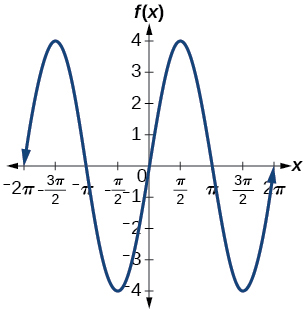amplitude: 4; period: $\text{\hspace{0.17em}}2\pi ;\text{\hspace{0.17em}}$ midline: $\text{\hspace{0.17em}}y=0;\text{\hspace{0.17em}}$ maximum $\text{\hspace{0.17em}}y=4\text{\hspace{0.17em}}$ occurs at $\text{\hspace{0.17em}}x=\frac{\pi }{2};\text{\hspace{0.17em}}$ minimum: $\text{\hspace{0.17em}}y=-4\text{\hspace{0.17em}}$ occurs at $\text{\hspace{0.17em}}x=\frac{3\pi }{2};\text{\hspace{0.17em}}$ one full period occurs from $\text{\hspace{0.17em}}x=0\text{\hspace{0.17em}}$ to $\text{\hspace{0.17em}}x=2\pi$

$f\left(x\right)=2\mathrm{cos}\text{\hspace{0.17em}}x$

$f\left(x\right)=\mathrm{cos}\left(2x\right)$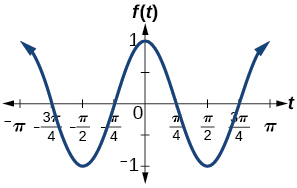amplitude: 1; period: $\text{\hspace{0.17em}}\pi ;\text{\hspace{0.17em}}$ midline: $\text{\hspace{0.17em}}y=0;\text{\hspace{0.17em}}$ maximum: $\text{\hspace{0.17em}}y=1\text{\hspace{0.17em}}$ occurs at $\text{\hspace{0.17em}}x=\pi ;\text{\hspace{0.17em}}$ minimum: $\text{\hspace{0.17em}}y=-1\text{\hspace{0.17em}}$ occurs at $\text{\hspace{0.17em}}x=\frac{\pi }{2};\text{\hspace{0.17em}}$ one full period is graphed from $\text{\hspace{0.17em}}x=0\text{\hspace{0.17em}}$ to $\text{\hspace{0.17em}}x=\pi$

$f\left(x\right)=2\text{\hspace{0.17em}}\mathrm{sin}\left(\frac{1}{2}x\right)$

$f\left(x\right)=4\text{\hspace{0.17em}}\mathrm{cos}\left(\pi x\right)$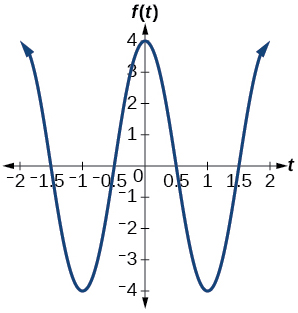amplitude: 4; period: 2; midline: $\text{\hspace{0.17em}}y=0;\text{\hspace{0.17em}}$ maximum: $\text{\hspace{0.17em}}y=4\text{\hspace{0.17em}}$ occurs at $\text{\hspace{0.17em}}x=0;\text{\hspace{0.17em}}$ minimum: $\text{\hspace{0.17em}}y=-4\text{\hspace{0.17em}}$ occurs at $\text{\hspace{0.17em}}x=1$

$f\left(x\right)=3\text{\hspace{0.17em}}\mathrm{cos}\left(\frac{6}{5}x\right)$

$y=3\text{\hspace{0.17em}}\mathrm{sin}\left(8\left(x+4\right)\right)+5$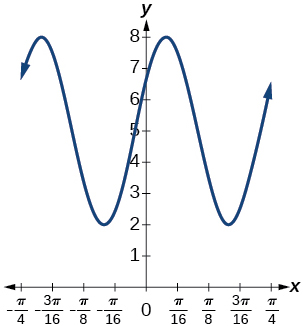amplitude: 3; period: $\text{\hspace{0.17em}}\frac{\pi }{4};\text{\hspace{0.17em}}$ midline: $\text{\hspace{0.17em}}y=5;\text{\hspace{0.17em}}$ maximum: $\text{\hspace{0.17em}}y=8\text{\hspace{0.17em}}$ occurs at $\text{\hspace{0.17em}}x=0.12;\text{\hspace{0.17em}}$ minimum: $\text{\hspace{0.17em}}y=2\text{\hspace{0.17em}}$ occurs at $\text{\hspace{0.17em}}x=0.516;\text{\hspace{0.17em}}$ horizontal shift: $\text{\hspace{0.17em}}-4;\text{\hspace{0.17em}}$ vertical translation 5; one period occurs from $\text{\hspace{0.17em}}x=0\text{\hspace{0.17em}}$ to $\text{\hspace{0.17em}}x=\frac{\pi }{4}$

$y=2\text{\hspace{0.17em}}\mathrm{sin}\left(3x-21\right)+4$

$y=5\text{\hspace{0.17em}}\mathrm{sin}\left(5x+20\right)-2$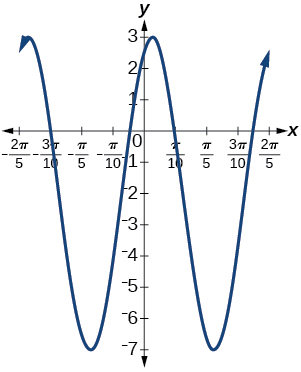amplitude: 5; period: $\text{\hspace{0.17em}}\frac{2\pi }{5};\text{\hspace{0.17em}}$ midline: $\text{\hspace{0.17em}}y=-2;\text{\hspace{0.17em}}$ maximum: $\text{\hspace{0.17em}}y=3\text{\hspace{0.17em}}$ occurs at $\text{\hspace{0.17em}}x=0.08;\text{\hspace{0.17em}}$ minimum: $\text{\hspace{0.17em}}y=-7\text{\hspace{0.17em}}$ occurs at $\text{\hspace{0.17em}}x=0.71;\text{\hspace{0.17em}}$ phase shift: $\text{\hspace{0.17em}}-4;\text{\hspace{0.17em}}$ vertical translation: $\text{\hspace{0.17em}}-2;\text{\hspace{0.17em}}$ one full period can be graphed on $\text{\hspace{0.17em}}x=0\text{\hspace{0.17em}}$ to $\text{\hspace{0.17em}}x=\frac{2\pi }{5}$

For the following exercises, graph one full period of each function, starting at $\text{\hspace{0.17em}}x=0.\text{\hspace{0.17em}}$ For each function, state the amplitude, period, and midline. State the maximum and minimum y -values and their corresponding x -values on one period for $\text{\hspace{0.17em}}x>0.\text{\hspace{0.17em}}$ State the phase shift and vertical translation, if applicable. Round answers to two decimal places if necessary.

$f\left(t\right)=2\mathrm{sin}\left(t-\frac{5\pi }{6}\right)$

$f\left(t\right)=-\mathrm{cos}\left(t+\frac{\pi }{3}\right)+1$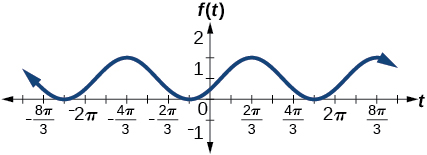amplitude: 1 ; period: $\text{\hspace{0.17em}}2\pi ;\text{\hspace{0.17em}}$ midline: $\text{\hspace{0.17em}}y=1;\text{\hspace{0.17em}}$ maximum: $\text{\hspace{0.17em}}y=2\text{\hspace{0.17em}}$ occurs at $\text{\hspace{0.17em}}x=2.09;\text{\hspace{0.17em}}$ maximum: $\text{\hspace{0.17em}}y=2\text{\hspace{0.17em}}$ occurs at $\text{\hspace{0.17em}}t=2.09;\text{\hspace{0.17em}}$ minimum: $\text{\hspace{0.17em}}y=0\text{\hspace{0.17em}}$ occurs at $\text{\hspace{0.17em}}t=5.24;\text{\hspace{0.17em}}$ phase shift: $\text{\hspace{0.17em}}-\frac{\pi }{3};\text{\hspace{0.17em}}$ vertical translation: 1; one full period is from $\text{\hspace{0.17em}}t=0\text{\hspace{0.17em}}$ to $\text{\hspace{0.17em}}t=2\pi$

$f\left(t\right)=4\mathrm{cos}\left(2\left(t+\frac{\pi }{4}\right)\right)-3$

$f\left(t\right)=-\mathrm{sin}\left(\frac{1}{2}t+\frac{5\pi }{3}\right)$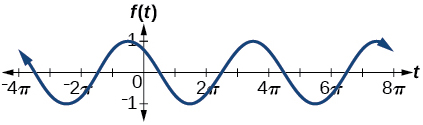amplitude: 1; period: $\text{\hspace{0.17em}}4\pi ;\text{\hspace{0.17em}}$ midline: $\text{\hspace{0.17em}}y=0;\text{\hspace{0.17em}}$ maximum: $\text{\hspace{0.17em}}y=1\text{\hspace{0.17em}}$ occurs at $\text{\hspace{0.17em}}t=11.52;\text{\hspace{0.17em}}$ minimum: $\text{\hspace{0.17em}}y=-1\text{\hspace{0.17em}}$ occurs at $\text{\hspace{0.17em}}t=5.24;\text{\hspace{0.17em}}$ phase shift: $\text{\hspace{0.17em}}-\frac{10\pi }{3};\text{\hspace{0.17em}}$ vertical shift: 0

$f\left(x\right)=4\mathrm{sin}\left(\frac{\pi }{2}\left(x-3\right)\right)+7$

Determine the amplitude, midline, period, and an equation involving the sine function for the graph shown in [link] .

amplitude: 2; midline: $\text{\hspace{0.17em}}y=-3;\text{\hspace{0.17em}}$ period: 4; equation: $\text{\hspace{0.17em}}f\left(x\right)=2\mathrm{sin}\left(\frac{\pi }{2}x\right)-3$

Determine the amplitude, period, midline, and an equation involving cosine for the graph shown in [link] .

Determine the amplitude, period, midline, and an equation involving cosine for the graph shown in [link] .

amplitude: 2; period: 5; midline: $\text{\hspace{0.17em}}y=3;\text{\hspace{0.17em}}$ equation: $\text{\hspace{0.17em}}f\left(x\right)=-2\mathrm{cos}\left(\frac{2\pi }{5}x\right)+3$

Determine the amplitude, period, midline, and an equation involving sine for the graph shown in [link] .

Determine the amplitude, period, midline, and an equation involving cosine for the graph shown in [link] .

amplitude: 4; period: 2; midline: $\text{\hspace{0.17em}}y=0;\text{\hspace{0.17em}}$ equation: $\text{\hspace{0.17em}}f\left(x\right)=-4\mathrm{cos}\left(\pi \left(x-\frac{\pi }{2}\right)\right)$

Determine the amplitude, period, midline, and an equation involving sine for the graph shown in [link] .

Determine the amplitude, period, midline, and an equation involving cosine for the graph shown in [link] .

amplitude: 2; period: 2; midline $\text{\hspace{0.17em}}y=1;\text{\hspace{0.17em}}$ equation: $\text{\hspace{0.17em}}f\left(x\right)=2\mathrm{cos}\left(\pi x\right)+1$

Determine the amplitude, period, midline, and an equation involving sine for the graph shown in [link] .

## Algebraic

For the following exercises, let $\text{\hspace{0.17em}}f\left(x\right)=\mathrm{sin}\text{\hspace{0.17em}}x.$

On $\text{\hspace{0.17em}}\left[0,2\pi \right),$ solve $\text{\hspace{0.17em}}f\left(x\right)=0.$

On $\text{\hspace{0.17em}}\left[0,2\pi \right),$ solve $\text{\hspace{0.17em}}f\left(x\right)=\frac{1}{2}.$

$\frac{\pi }{6},\frac{5\pi }{6}$

Evaluate $\text{\hspace{0.17em}}f\left(\frac{\pi }{2}\right).$

On $\text{\hspace{0.17em}}\left[0,2\pi \right),f\left(x\right)=\frac{\sqrt{2}}{2}.\text{\hspace{0.17em}}$ Find all values of $\text{\hspace{0.17em}}x.$

$\frac{\pi }{4},\frac{3\pi }{4}$

On $\text{\hspace{0.17em}}\left[0,2\pi \right),$ the maximum value(s) of the function occur(s) at what x -value(s)?

On $\text{\hspace{0.17em}}\left[0,2\pi \right),$ the minimum value(s) of the function occur(s) at what x -value(s)?

$\frac{3\pi }{2}$

Show that $\text{\hspace{0.17em}}f\left(-x\right)=-f\left(x\right).\text{\hspace{0.17em}}$ This means that $\text{\hspace{0.17em}}f\left(x\right)=\mathrm{sin}\text{\hspace{0.17em}}x\text{\hspace{0.17em}}$ is an odd function and possesses symmetry with respect to ________________.

For the following exercises, let $\text{\hspace{0.17em}}f\left(x\right)=\mathrm{cos}\text{\hspace{0.17em}}x.$

On $\text{\hspace{0.17em}}\left[0,2\pi \right),$ solve the equation $\text{\hspace{0.17em}}f\left(x\right)=\mathrm{cos}\text{\hspace{0.17em}}x=0.$

$\frac{\pi }{2},\frac{3\pi }{2}$

On $\text{\hspace{0.17em}}\left[0,2\pi \right),$ solve $\text{\hspace{0.17em}}f\left(x\right)=\frac{1}{2}.$

On $\text{\hspace{0.17em}}\left[0,2\pi \right),$ find the x -intercepts of $\text{\hspace{0.17em}}f\left(x\right)=\mathrm{cos}\text{\hspace{0.17em}}x.$

$\frac{\pi }{2},\frac{3\pi }{2}$

On $\text{\hspace{0.17em}}\left[0,2\pi \right),$ find the x -values at which the function has a maximum or minimum value.

On $\text{\hspace{0.17em}}\left[0,2\pi \right),$ solve the equation $\text{\hspace{0.17em}}f\left(x\right)=\frac{\sqrt{3}}{2}.$

$\frac{\pi }{6},\frac{11\pi }{6}$

## Technology

Graph $\text{\hspace{0.17em}}h\left(x\right)=x+\mathrm{sin}\text{\hspace{0.17em}}x\text{\hspace{0.17em}}$ on $\text{\hspace{0.17em}}\left[0,2\pi \right].\text{\hspace{0.17em}}$ Explain why the graph appears as it does.

Graph $\text{\hspace{0.17em}}h\left(x\right)=x+\mathrm{sin}\text{\hspace{0.17em}}x\text{\hspace{0.17em}}$ on $\text{\hspace{0.17em}}\left[-100,100\right].\text{\hspace{0.17em}}$ Did the graph appear as predicted in the previous exercise?

The graph appears linear. The linear functions dominate the shape of the graph for large values of $\text{\hspace{0.17em}}x.$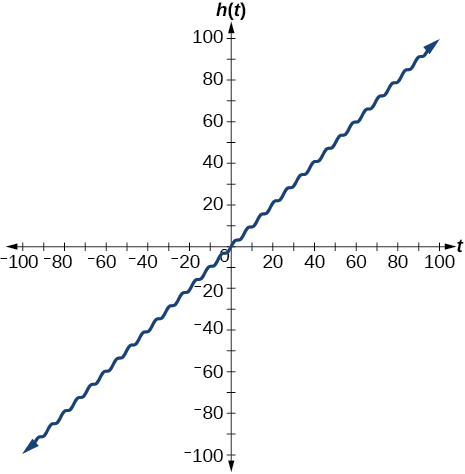Graph $\text{\hspace{0.17em}}f\left(x\right)=x\text{\hspace{0.17em}}\mathrm{sin}\text{\hspace{0.17em}}x\text{\hspace{0.17em}}$ on $\text{\hspace{0.17em}}\left[0,2\pi \right]\text{\hspace{0.17em}}$ and verbalize how the graph varies from the graph of $\text{\hspace{0.17em}}f\left(x\right)=\mathrm{sin}\text{\hspace{0.17em}}x.$

Graph $\text{\hspace{0.17em}}f\left(x\right)=x\text{\hspace{0.17em}}\mathrm{sin}\text{\hspace{0.17em}}x\text{\hspace{0.17em}}$ on the window $\text{\hspace{0.17em}}\left[-10,10\right]\text{\hspace{0.17em}}$ and explain what the graph shows.

The graph is symmetric with respect to the y -axis and there is no amplitude because the function is not periodic.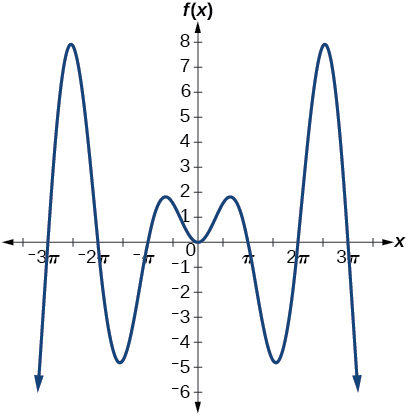Graph $\text{\hspace{0.17em}}f\left(x\right)=\frac{\mathrm{sin}\text{\hspace{0.17em}}x}{x}\text{\hspace{0.17em}}$ on the window $\text{\hspace{0.17em}}\left[-5\pi ,5\pi \right]\text{\hspace{0.17em}}$ and explain what the graph shows.

## Real-world applications

A Ferris wheel is 25 meters in diameter and boarded from a platform that is 1 meter above the ground. The six o’clock position on the Ferris wheel is level with the loading platform. The wheel completes 1 full revolution in 10 minutes. The function $\text{\hspace{0.17em}}h\left(t\right)\text{\hspace{0.17em}}$ gives a person’s height in meters above the ground t minutes after the wheel begins to turn.

1. Find the amplitude, midline, and period of $\text{\hspace{0.17em}}h\left(t\right).$
2. Find a formula for the height function $\text{\hspace{0.17em}}h\left(t\right).$
3. How high off the ground is a person after 5 minutes?
1. Amplitude: 12.5; period: 10; midline: $\text{\hspace{0.17em}}y=13.5;$
2. $h\left(t\right)=12.5\mathrm{sin}\left(\frac{\pi }{5}\left(t-2.5\right)\right)+13.5;$
3. 26 ft

#### Questions & Answers

write down the polynomial function with root 1/3,2,-3 with solution
if A and B are subspaces of V prove that (A+B)/B=A/(A-B)
write down the value of each of the following in surd form a)cos(-65°) b)sin(-180°)c)tan(225°)d)tan(135°)
Prove that (sinA/1-cosA - 1-cosA/sinA) (cosA/1-sinA - 1-sinA/cosA) = 4
what is the answer to dividing negative index
In a triangle ABC prove that. (b+c)cosA+(c+a)cosB+(a+b)cisC=a+b+c.
give me the waec 2019 questions
the polar co-ordinate of the point (-1, -1)
prove the identites sin x ( 1+ tan x )+ cos x ( 1+ cot x )= sec x + cosec x
tanh`(x-iy) =A+iB, find A and B
B=Ai-itan(hx-hiy)
Rukmini
what is the addition of 101011 with 101010
If those numbers are binary, it's 1010101. If they are base 10, it's 202021.
Jack
extra power 4 minus 5 x cube + 7 x square minus 5 x + 1 equal to zero
the gradient function of a curve is 2x+4 and the curve passes through point (1,4) find the equation of the curve
1+cos²A/cos²A=2cosec²A-1
test for convergence the series 1+x/2+2!/9x3ByBy RhodesByBy Rhodes